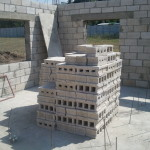## How to Calculate the First Moment of AreaIn structural engineering applications, the first moment of area is a calculation that is used to determine shear stress distributions. It is a relatively simple concept which involves the moment of a surface around an axis. Where: Qx = first moment around the x axis (ft3 or m3) Qy = first moment around the y […]